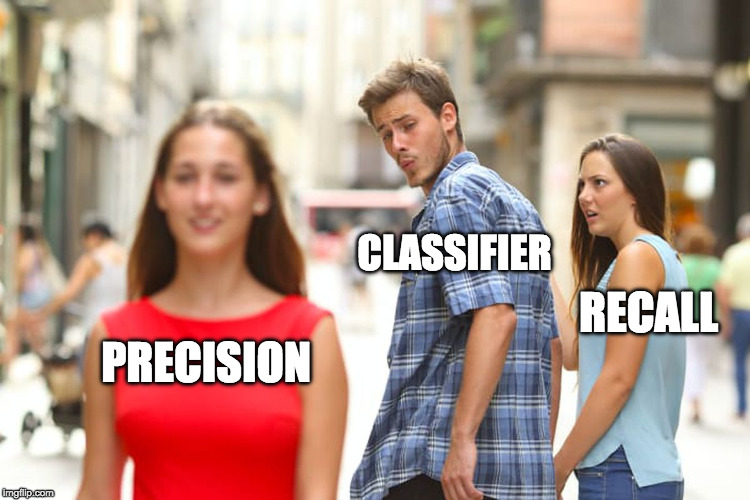## Curriculum Vitae for Data Scientists

Applying for a data scientist job offer? Tired of writing the same old curriculum vitae?

## Intro to OpenCV with Python

To work with OpenCV from python, you need to install it first:

`pip install opencv-python`

After we import cv2 we can directly work with images like so:

```import cv2
```

## Too confused of the confusion matrix?

Let me bring some clarity into this topic!

## numpy random choice

With numpy you can easily create test data with random_integers and randint.

```numpy.random.randint(low, high=None, size=None, dtype='l')
numpy.random.random_integers(low, high=None, size=None)```

random_integers includes the high boundary while randint does not. Continue reading “numpy random choice”

## Classification: Precision and Recall

In the realms of Data Science you’ll encounter sooner or the later the terms “Precision” and “Recall”. But what do they mean?## Clarification

Living together with little kids You very often run into classification issues:

My daughter really likes dogs, so seeing a dog is something positive. When she sees a normal dog e.g. a Labrador and proclaims: “Look, there is a dog!”

That’s a True Positive (TP) Continue reading “Classification: Precision and Recall”

## UD120 – Intro to Machine Learning

One part of my bucket list for 2018 is finishing the Udacity Course UD120: Intro to Machine Learning.

The host of this course are Sebastian Thrun, ex-google-X and founder of Udacity and Katie Malone, creator of the Linear digressions podcast.

The course consists of 17 lessons. Every lesson has a couple of hours of video and lots and lots of quizzes in it. Continue reading “UD120 – Intro to Machine Learning”

## Lesson 3: Support Vector Machines

The term Support Vector Machines or SVM is a bit misleading. It is just a name for a very clever algorithm invented by two Russians. in the 1960s. SVMs are used for classification and regression.

SVM do that by finding a hyperplane between two classes of data which separates both classes best. Continue reading “Lesson 3: Support Vector Machines”

## Linear Algebra with numpy – Part 1

Numpy is a package for scientific computing in Python.

`import numpy as np`

The most important data structure is ndarray, which is short for n-dimensional array.

You can convert a list to an numpy array with the array-method

```my_list = [1, 2, 3, 4]
my_array = np.array(my_list)```

You can also convert an array back to a list with Continue reading “Linear Algebra with numpy – Part 1”

## The Normal Distribution

Diving deeper into data science I started to brush up my knowledge about math especially statistics.

## The Mother of all DistributionsThe normal distribution was formulated by Carl Friedrich Gauß in 18XX and can be implemented in Python like the following :

```def normal_distribution(x, mu=0, sigma=1):
sqrt_two_pi = math.sqrt(2*math.pi)
return math.exp(-(x-mu)**2 / 2 / sigma**2) / sqrt_two_pi * sigma```